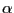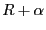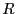Next: Condition number Up: Possible parameters values for Previous: Approximation of the set   Contents

Largest square enclosed in the regions

This second procedure allows to compute the largest square (up to a pre-defined accuracy) that is enclosed in the region. This largest box can clearly be obtained from the result of the previous algorithm but this weaker procedure will be faster.

The principle is to have a set of boxes whose first element is a range for the center of the box and second element is a possible value for the length of the half-edge of the square. The main algorithm will test if for a given box the center may be a candidate to be the center of a square of half-edge(whereis the current optimum) using some heuristics and if the answer is positive will compute the minimal and maximal root of the polynomials defined by this square using a secondary algorithm. If these values are compatible with the bound the current optimum will be updated.

int ALIAS_Geometry_Carre(int Degree,int Nb_Parameter,
INTERVAL_VECTOR (* TheCoeff)(INTERVAL_VECTOR &),
int Nb_Constraints,INTEGER_VECTOR &Type_Eq,INTEGER_VECTOR &Imperatif,
int (* TheMatrix)(INTERVAL_VECTOR &, INTERVAL_MATRIX &),
int Has_Matrix,
INTERVAL_VECTOR (* IntervalFunction)(int,int,INTERVAL_VECTOR &),
INTERVAL & TheDomain,INTERVAL_VECTOR & TheDomain_Parameter,
int Nb_Points,int Use_Solve,int rand,
int Iteration_Geometry,int Iteration_Polynom,
double Accuracy_Variable,double Accuracy,double Accuracy_Geometry,
double Accuracy_Polynom,INTERVAL_VECTOR &Solution,double *Seuil,
int (* Solve_Poly)(double *, int *,double *),
int (* Simp_Proc)(INTERVAL_VECTOR &),
int (* Simp_Proc_Pol)(INTERVAL_VECTOR &))
The arguments are the same than for the previous procedure except for:
• Imperatif: an array of integer. If there are constraints that are imperative, meaning that the polynomial cannot be evaluated if they are not satisfied (for example the constraints is that some term is positive as in the coefficients of the polynomial this term appears as a square root) you may set the corresponding integer to 1. If this array has dimension 0 all the constraint will supposed to be not imperative
• Iteration_Geometry: the number of boxes that may be used by the main algorithm
• Iteration_Polynom: the number of boxes that may be used by the secondary algorithm
• Accuracy_Geometry: the value of• Accuracy_Polynom: the accuracy used for the secondary algorithm. Note that this parameter play a role not only on the computation time but also on the bound for the root. Indeed the algorithm will verify that in the square there is no polynomial with a root lower than Seuil+Accuracy_Polynom or larger than Seuil-Accuracy_Polynom
• Solution: the point interval is the center of the largest square while the second is the value of the half-edge
• Simp_Proc: a simplification procedure used only in the main algorithm. The flag ALIAS_Simp_Main is set to 1 when this procedure is called right after the bisection process.
• Simp_Proc_Polynom: a simplification procedure used only in the secondary algorithm

This procedure will return:

• -1000: error in the Single_Bisection flag that should be between 0 and 5
• -4: error in Type_Eq
• -3: the lowest root of all the polynomials is greater than Seuil
• -2: the highest root of all the polynomials is lower than Seuil
• -1: the algorithm has failed
• 0: the result is not guaranteed
• 1:the result is guaranteed

During the calculation the flag ALIAS_Has_OptimumG will be set to 1 as soon as an optimum is found: the center of the current optimal geometry is the mid point of the interval vector ALIAS_Vector_OptimumG while is edge is in the interval ALIAS_OptimumG.

The secondary algorithm uses the flag Single_BisectionG and Reverse_StrorageG that play the same role than Single_Bisection and Reverse_Storage in the general solving algorithms (see sections 2.3.1.3 and 2.3.1.2).Next: Condition number Up: Possible parameters values for Previous: Approximation of the set   Contents
Jean-Pierre Merlet 2012-12-20